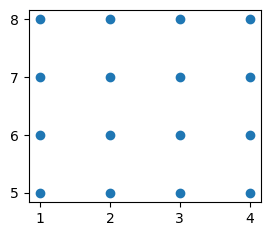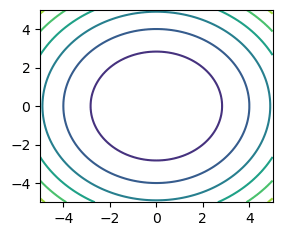search
Search
menu search toc more_vert
Guest 0reps
Thanks for the thanks!
close
account_circle
Profile
exit_to_app
Sign out
help Ask a question
search
keyboard_voice
close
Searching Tips
Search for a recipe:
"Creating a table in MySQL"
Search for an API documentation: "@append"
Search for code: "!dataframe"
Apply a tag filter: "#python"
Useful Shortcuts
/ to open search panel
Esc to close search panel
to navigate between search results
d to clear all current filters
Enter to expand content previewDoc SearchCode Search BetaSORRY NOTHING FOUND!
mic
Start speaking...Voice search is only supported in Safari and Chrome.
Shrink
Navigate to
A
A
brightness_medium
share
arrow_backShareTwitterFacebook

# NumPy | meshgrid method

NumPy
chevron_right
Documentation
schedule Jul 1, 2022
Last updated
local_offer PythonNumPy
Tags
expand_more

Numpy's `meshgrid(~)` method returns a grid that is useful in plotting contour plots of 3D graphs. Since it is difficult to explain in words what this method does, consult the examples below.

# Parameters

1. `x1` | `array-like`

The input array to make a grid out of.

2. `x2` | `array-like` | `optional`

If you want a 2D grid, then this is required.

* Note that you could add in more arrays (i.e. x3, x4,...) if desired.

3. `sparse` | `boolean` | `optional`

Whether to return a sparse grid. Set this to `True` if you're dealing with large volumes of data that cannot be fit into memory. By default, `sparse=False`.

4. `copy` | `boolean` | `optional`

If `True`, then a new Numpy array is created and returned. If `False`, then view is returned so as to save memory. By default, `copy=True`.

# Return value

If only `x1` is specified, then a Numpy array is returned. Otherwise, a tuple of Numpy arrays will be returned.

# Examples

## Visualising the mesh grid

Let us create a 2D mesh grid:

``` x = [1,2,3,4]y = [5,6,7,8]xx, yy = np.meshgrid(x, y) ```

Let's unveil the content of the returned arrays:

``` print(xx) [[1 2 3 4] [1 2 3 4] [1 2 3 4] [1 2 3 4]] ```

Here's `yy`:

``` print(yy) [[5 5 5 5] [6 6 6 6] [7 7 7 7] [8 8 8 8]] ```

Using Matplotlib, we can graph them like so:

``` plt.scatter(xx, yy)plt.show() ```

This gives us the following:## Drawing a contour plot

Let's now talk about the practical benefits of having these data-points. They come in handy when we draw contour plots of 3D functions.

Here's an example:

``` def f(x, y): return x**2 + y **2 ```

We can draw the contour like so:

``` x = np.linspace(-5, 5, 100)y = np.linspace(-5, 5, 100)xx, yy = np.meshgrid(x, y)zz = f(xx, yy)plt.contour(xx, yy, zz)plt.show() ```

This gives us the following contour plot:mail